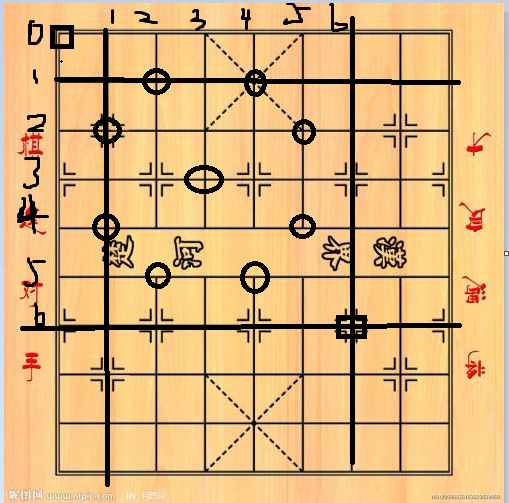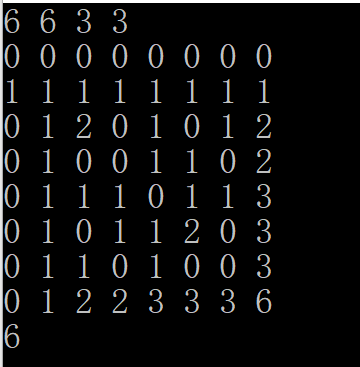#include<iostream>
using namespace std;
long long horse,map;
void Mark(long long x,long long y) //马能跳的地方
{//因为数组开的足够大所以，不用判断会不会向下和右越界
horse[x][y]=1;
if(x-2>=0||y>=1)
horse[x-2][y-1]=1;
if(y-2>=0&&x>=1)
horse[x-1][y-2]=1;
if(y-2>=0)
horse[x+1][y-2]=1;
if(y-1>=0)
horse[x+2][y-1]=1;
if(x-2>=0)
horse[x-2][y+1]=1;
if(x-1>=0)
horse[x-1][y+2]=1;
horse[x+2][y+1]=1;
horse[x+1][y+2]=1;
}
int main()
{
long long x,y,n,m;
cin>>n>>m>>x>>y;
Mark(x,y);
map=1;//我把它叫做：状态转移方程的入口
for(int i=1;i<=n+1;i++)//怕负数，把数组全往右下移动1个单位
{
for(int j=1;j<=m+1;j++)
{
map[i][j]=map[i-1][j]+map[i][j-1];//状态转移方程
if(horse[i-1][j-1])map[i][j]=0;
}
}
cout<<map[n+1][m+1];//往右下移动一个位置咯
return 0;
}
View Codeposted on 2018-05-15 22:12  阙广壬辰  阅读(267)  评论(0编辑  收藏  举报